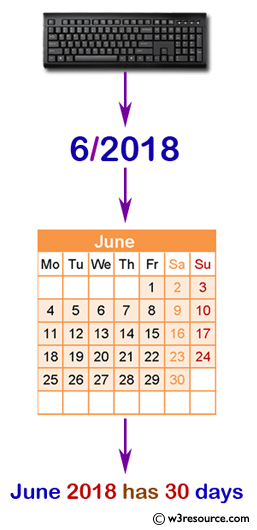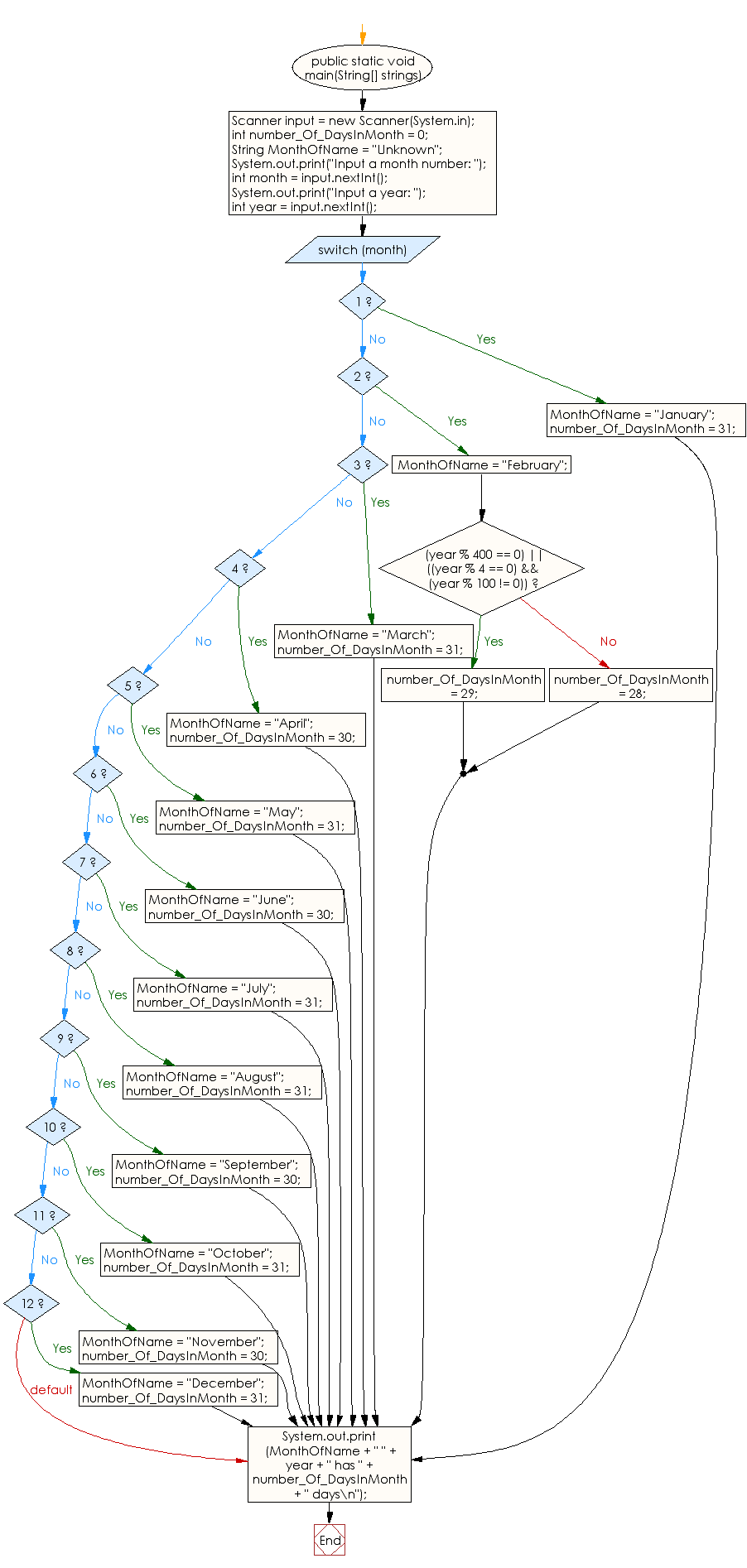﻿ Java - Find the number of days in a month

# Java: Find the number of days in a month

## Java Conditional Statement: Exercise-7 with Solution

Write a Java program to find the number of days in a month.

Test Data
Input a month number: 2
Input a year: 2016

Pictorial Presentation:Sample Solution:

Java Code:

``````import java.util.Scanner;
public class Exercise7 {

public static void main(String[] strings) {

Scanner input = new Scanner(System.in);

int number_Of_DaysInMonth = 0;
String MonthOfName = "Unknown";

System.out.print("Input a month number: ");
int month = input.nextInt();

System.out.print("Input a year: ");
int year = input.nextInt();

switch (month) {
case 1:
MonthOfName = "January";
number_Of_DaysInMonth = 31;
break;
case 2:
MonthOfName = "February";
if ((year % 400 == 0) || ((year % 4 == 0) && (year % 100 != 0))) {
number_Of_DaysInMonth = 29;
} else {
number_Of_DaysInMonth = 28;
}
break;
case 3:
MonthOfName = "March";
number_Of_DaysInMonth = 31;
break;
case 4:
MonthOfName = "April";
number_Of_DaysInMonth = 30;
break;
case 5:
MonthOfName = "May";
number_Of_DaysInMonth = 31;
break;
case 6:
MonthOfName = "June";
number_Of_DaysInMonth = 30;
break;
case 7:
MonthOfName = "July";
number_Of_DaysInMonth = 31;
break;
case 8:
MonthOfName = "August";
number_Of_DaysInMonth = 31;
break;
case 9:
MonthOfName = "September";
number_Of_DaysInMonth = 30;
break;
case 10:
MonthOfName = "October";
number_Of_DaysInMonth = 31;
break;
case 11:
MonthOfName = "November";
number_Of_DaysInMonth = 30;
break;
case 12:
MonthOfName = "December";
number_Of_DaysInMonth = 31;
}
System.out.print(MonthOfName + " " + year + " has " + number_Of_DaysInMonth + " days\n");
}
}
```
```

Sample Output:

```Input a month number: 2
Input a year: 2016
February 2016 has 29 days
```

Flowchart:Java Code Editor:

What is the difficulty level of this exercise?

Test your Programming skills with w3resource's quiz.

﻿

## Java: Tips of the Day

IsPowerOfTwo

Checks if a value is positive power of two.

To understand how it works let's assume we made a call IsPowerOfTwo(4).

As value is greater than 0, so right side of the && operator will be evaluated.

The result of (~value + 1) is equal to value itself. ~100 + 001 => 011 + 001 => 100. This is equal to value.

The result of (value & value) is value. 100 & 100 => 100.

This will value the expression to true as value is equal to value.

```public static boolean isPowerOfTwo(final int value) {
return value > 0 && ((value & (~value + 1)) == value);
}
```

Ref: https://bit.ly/3sA5d4I

We are closing our Disqus commenting system for some maintenanace issues. You may write to us at reach[at]yahoo[dot]com or visit us at Facebook